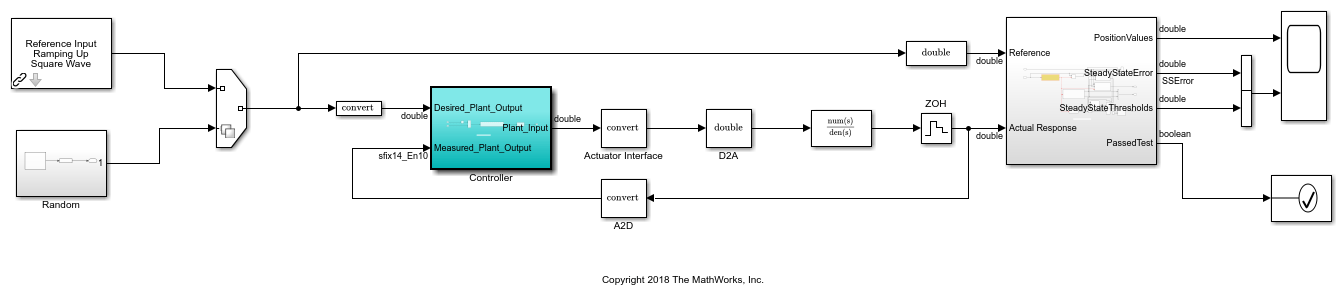# Optimize Data Types Using Multiple Simulation Scenarios

This example shows how to create multiple simulation scenarios, and use the scenarios to optimize the fixed-point data types of a system.

Open the model. In this example, you optimize the data types of the Controller subsystem. The model is set up to use either a ramp input, or a random input. The model uses an Assertion block rather than using signal tolerances to verify the numerical behavior of the fixed-point implementation. For more information, see Specify Behavioral Constraints.

```model = 'ex_controllerHarness'; open_system(model); ```### Create the Simulation Scenarios

Create a `Simulink.SimulationInput` object that contains the different scenarios. Use both the ramp input as well as four different seeds for the random input.

```si = Simulink.SimulationInput.empty(5, 0); % scan through 4 different seeds for the random input rng(1); seeds = randi(1e6, [1 4]); for sIndex = 1:length(seeds) si(sIndex) = Simulink.SimulationInput(model); si(sIndex) = si(sIndex).setVariable('SOURCE', 2); % SOURCE == 2 corresponds to the random input si(sIndex) = si(sIndex).setBlockParameter([model '/Random/uniformRandom'], 'Seed', num2str(seeds(sIndex))); % scan through the seeds si(sIndex) = si(sIndex).setUserString(sprintf('random_%i', seeds(sIndex))); end % setting SOURCE == 1 corresponds to the ramp input si(5) = Simulink.SimulationInput(model); si(5) = si(5).setVariable('SOURCE', 1); si(5) = si(5).setUserString('Ramp'); ```

### Specify Fixed-Point Optimization Options

To specify options for the optimization, such as the number of iterations and method for range collection, use the `fxpOptimizationOptions` object. This example uses derived range analysis to collect ranges for the system.

```options = fxpOptimizationOptions('MaxIterations', 3e2, 'Patience', 50); options.AdvancedOptions.PerformNeighborhoodSearch = false; % use derived range analysis for range collection options.AdvancedOptions.UseDerivedRangeAnalysis = true ```
```options = fxpOptimizationOptions with properties: MaxIterations: 300 MaxTime: 600 Patience: 50 Verbosity: High AllowableWordLengths: [2 3 4 5 6 7 8 9 10 11 12 13 14 15 16 17 18 19 ... ] ObjectiveFunction: BitWidthSum UseParallel: 0 Advanced Options AdvancedOptions: [1x1 DataTypeOptimization.AdvancedFxpOptimizationOptions] ```

Specify the simulation input objects as simulation scenarios in the advanced options.

```options.AdvancedOptions.SimulationScenarios = si; ```

### Run Optimization and Explore the Results

During the optimization, the software derives ranges for all simulation scenarios specified in the advanced options. The software verifies solutions against each simulation input scenario.

```result = fxpopt(model, [model '/Controller'], options) ```
``` + Starting data type optimization... + Checking for unsupported constructs. + Preprocessing + Modeling the optimization problem - Constructing decision variables + Running the optimization solver - Evaluating new solution: cost 496, does not meet the behavioral constraints. - Evaluating new solution: cost 976, does not meet the behavioral constraints. - Evaluating new solution: cost 1936, meets the behavioral constraints. - Updated best found solution, cost: 1936 + Optimization has finished. + Fixed-point implementation that satisfies the behavioral constraints found. The best found solution is applied on the model. - Total cost: 1936 - Use the explore method of the result to explore the implementation. result = OptimizationResult with properties: Model: 'ex_controllerHarness' SystemUnderDesign: 'ex_controllerHarness/Controller' FinalOutcome: 'Fixed-point implementation that satisfies the behavioral constraints found. The best found solution is applied on the model.' OptimizationOptions: [1x1 fxpOptimizationOptions] Solutions: [1x1 DataTypeOptimization.OptimizationSolution] ```

You can explore each solution as it compares to each simulation scenario you defined. Explore the best found solution and view it with the ramp simulation input. The ramp input is simulation scenario five.

```solutionIndex = 1; % get the best found solution scenarioIndex = 5; % get the 5th scenario (ramp) solution = explore(result, solutionIndex, scenarioIndex); ```